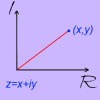#### You may also like### Thousand Words

Here the diagram says it all. Can you find the diagram?### A Brief Introduction to Complex Numbers

In this problem, we define complex numbers and invite you to explore what happens when you add and multiply them.### A Brief Introduction to the Argand Diagram

Complex numbers can be represented graphically using an Argand diagram. This problem explains more...

# Complex Squares

##### Age 16 to 18Challenge Level

You may find it useful to try A Brief Introduction to Complex Numbers and A Brief Introduction to the Argand Diagram before tackling this problem.

Any complex number $z=a+ib$ can be represented as a point $(a, b)$ on the Argand diagram.

What is special about complex numbers which square to give a real number?
Given any real number $x$, is there always a complex number that squares to give $x$?
Represent your findings on the Argand diagram.

Find some complex numbers which square to an imaginary number.
What can you say about such complex numbers?
Given any imaginary number, is there always a complex number that squares to give that number?
Represent your findings on the Argand diagram.

Explore the effects of squaring on other complex numbers as they are represented on the Argand diagram. You may wish to use GeoGebra.

Can you find any complex numbers which square to give the complex numbers you found in the first two parts of the problem?

Send us your thoughts on squaring complex numbers, any conjectures that arise, and any explanations for what you find.